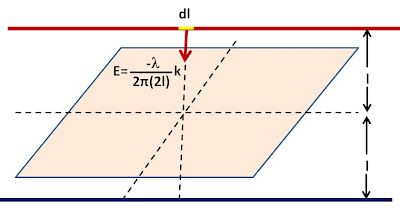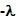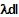## Wednesday, November 10, 2010

### Irodov Problem 3.60Based on the method of images we can replace the infinite conducting plane by an infintely long charged wire of chargeper unit length. As seen from Problem 3.22, the electric field due to the negively charged wire at a distance 2l will be,

Consider an infintesimally small section of the positively charged wire of length dl. The charge contained in this infinitesimally small section of wire will be. Thus, the force acting on this infinitesiamlly small section of the wire will be,

Based on problem 3.22, the electric field at a location on the conductor that is x untis from the conductor (as shown in the figure) is given by,

Similar to problem 3.59, if the charge density induced in the conductor at this location is given by, then,

#### 1 comment:

1.in the fifth image from the start,
dE should be E